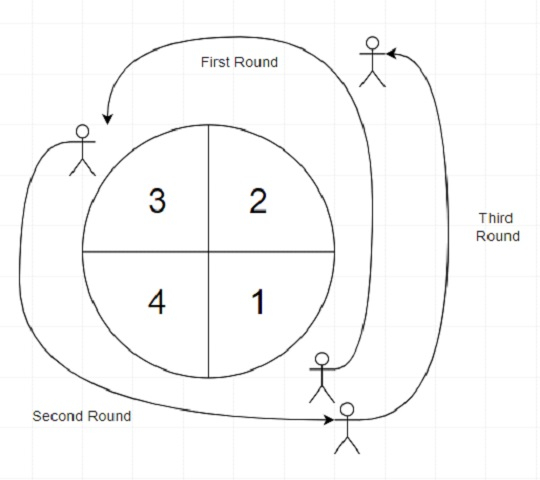# Program to find most visited sector in a circular track using Python

PythonServer Side ProgrammingProgramming

Suppose we have a number n and an array called rounds. We have a circular track which consists of n different sectors labeled from 1 to n. Now consider a race will be held on this track, the race consists of m different rounds. The ith round starts at sector rounds[i - 1] and ends at sector rounds[i]. For example, if the round 1 starts at sector rounds and ends at sector rounds. So we have to find the most visited sectors sorted in ascending order. (The track numbers are in ascending order of sector numbers in the counter-clockwise direction)

So, if the input is like n = 4 rounds = [1,3,1,2], then the output will be [1, 2]because the race starts from sector 1. The order of the visited sectors is as follows: [1,2,3 (end of first round),4,1 (end of second round), 2 (end of 3rd round)]. Here both of the sectors 1 and 2 are visited twice and they are the most visited sectors. And sectors 3 and 4 are visited only once.

To solve this, we will follow these steps −

• d := a new map

• for j in range 1 to n, do

• d[j] := 0

• d[rounds] := 1

• for i in range 1 to size of rounds - 1, do

• if rounds[i] > rounds[i-1], then

• for j in range rounds[i-1]+1 to rounds[i]+1, do

• d[j] := d[j] + 1

• otherwise,

• for j in range rounds[i-1]+1 to n, do

• d[j] := d[j] + 1

• for j in range 1 to rounds[i], do

• d[j] := d[j] + 1

• curr := d[rounds]

• out := [rounds]

• for i in range 1 to n, do

• if i is not same as rounds, then

• if d[i] > curr, then

• curr := d[i]

• out := [i]

• otherwise when d[i] is same as curr, then

• append i with out

• return out after sorting

## Example (Python)

Let us see the following implementation to get better understanding −

Live Demo

def solve(n, rounds):
d = {}
for j in range(1,n+1):
d[j] = 0
d[rounds] = 1
for i in range(1, len(rounds)):
if rounds[i] > rounds[i-1]:
for j in range(rounds[i-1]+1, rounds[i]+1):
d[j] += 1
else:
for j in range(rounds[i-1]+1,n+1):
d[j] += 1
for j in range(1,rounds[i]+1):
d[j] += 1

curr = d[rounds]
out = [rounds]
for i in range(1,n+1):
if i != rounds:
if d[i] > curr:
curr = d[i]
out = [i]
elif d[i] == curr:
out = out + [i]
return(sorted(out))

n = 4
rounds = [1,3,1,2]
print(solve(n, rounds))

## Input

4, [1,3,1,2]

## Output

[1, 2]